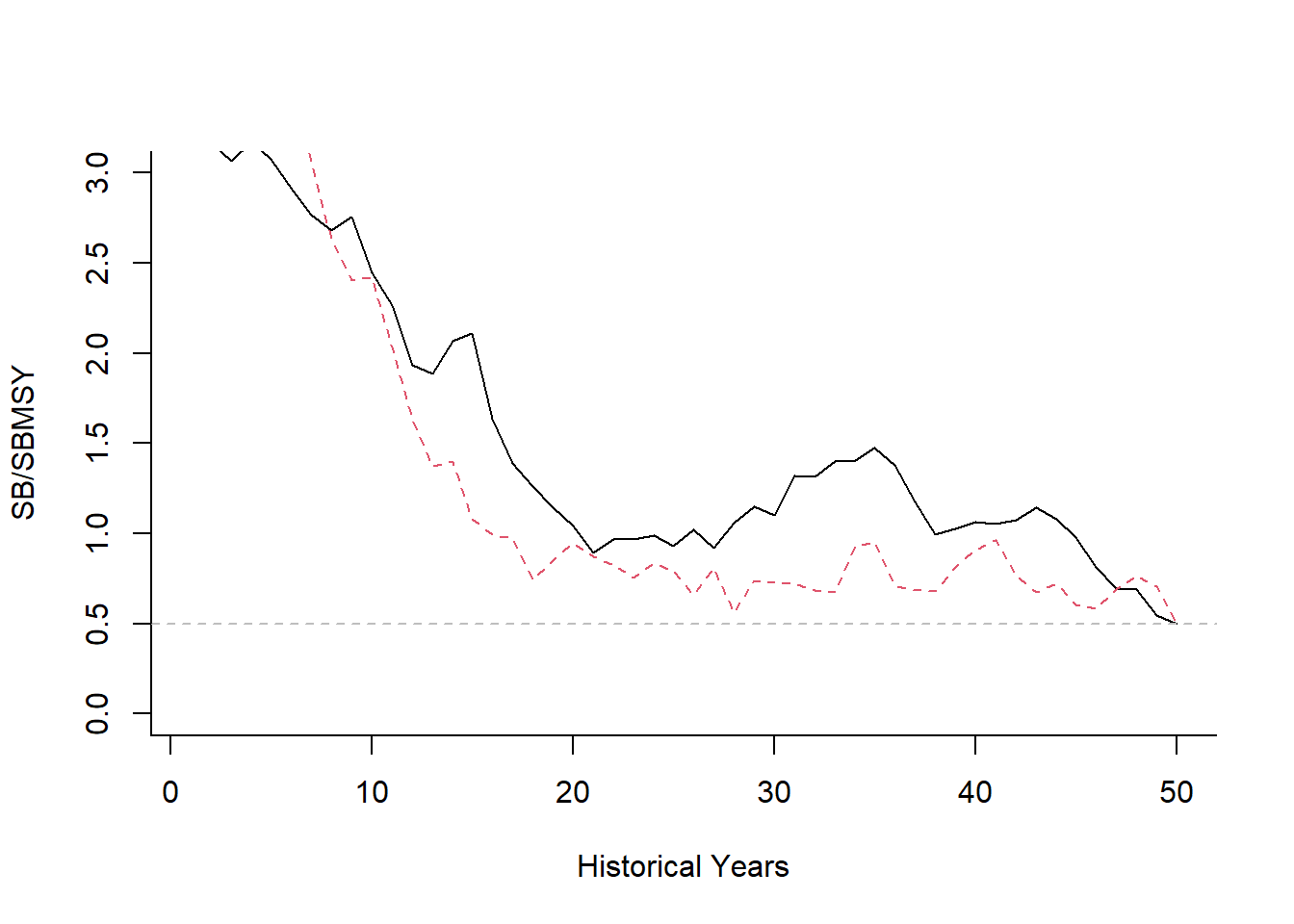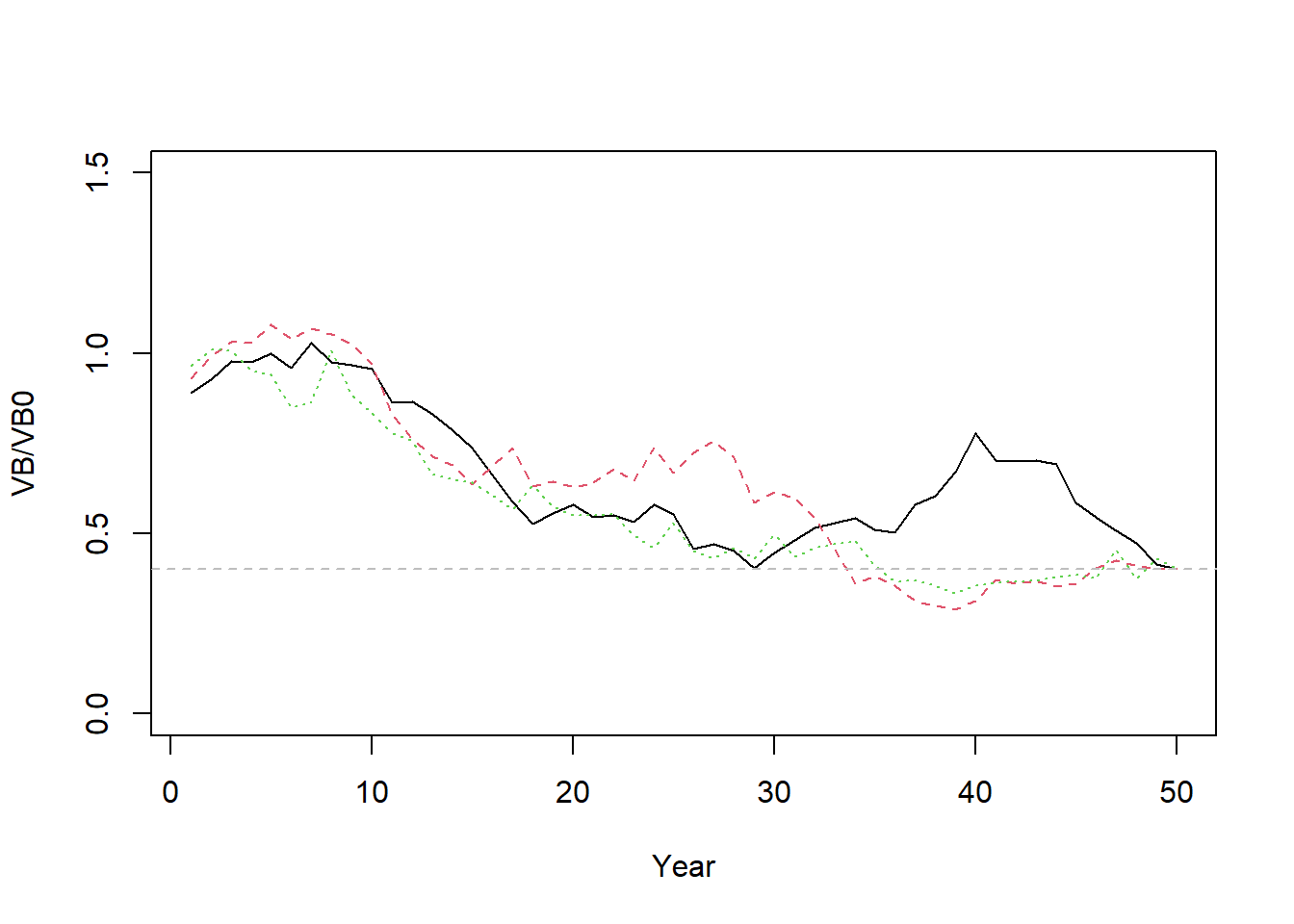## Setting Depletion at a fraction of SBMSY or VB0

Relative reference points such as $\frac{B_\text{MSY}}{B_0}$, and $\frac{SB_\text{MSY}}{SB_0}$ are calculated relative to the unfished biomass described in the previous section. This means that it is relatively straightforward to initialize the simulation model at a fraction of $\text{SB}_\text{MSY}$ rather than $\text{SB}_0$.

#### Depletion as fraction of SBMSY

For example, suppose you wish to set the depletion in the final historical year at $0.5SB_\text{MSY}$:

OM <- testOM; OM@nsim <- 2
# need to populate OM@cpars$D so that random samples are the same OM@cpars$D <- runif(OM@nsim, OM@D, OM@D)
Hist <- runMSE(OM, Hist=TRUE, silent=TRUE)
# update Depletion to 0.5 BMSY using cpars
OM@cpars$D <- 0.5 * Hist@Ref$ReferencePoints$SSBMSY_SSB0 Hist <- runMSE(OM, Hist=TRUE, silent=TRUE) matplot(t(apply(Hist@TSdata$SBiomass, 1:2, sum)/Hist@Ref$ReferencePoints$SSBMSY),
type="l", ylim=c(0,3),
ylab="SB/SBMSY", xlab="Historical Years", bty="l")
abline(h=0.5, lty=2, col="gray")#### Depletion as fraction of VB0

The model can be modified to optimize for depletion in terms of average unfished vulnerable biomass ($\text{VB}_0$) rather than spawning biomass using the control Custom Parameters feature:

OM <- testOM
# switch to depletion in terms of VB0
OM@cpars$control$D <- 'VB'

# set depletion
OM@cpars$D <- rep(0.4, OM@nsim) Hist <- Simulate(OM,silent=TRUE) # VB time-series VB <- apply(Hist@TSdata$VBiomass, 1:2, sum)

matplot(t(VB/matrix(Hist@Ref$ReferencePoints$VB0, nrow=OM@nsim, ncol=OM@nyears)),
type="l", xlab="Year", ylab="VB/VB0", ylim=c(0,1.5))
abline(h=0.4, lty=2, col="gray")